Courses

# Frequency and Temperature Dependence of Complex Elastic Modulus Civil Engineering (CE) Notes | EduRev

## Civil Engineering (CE) : Frequency and Temperature Dependence of Complex Elastic Modulus Civil Engineering (CE) Notes | EduRev

The document Frequency and Temperature Dependence of Complex Elastic Modulus Civil Engineering (CE) Notes | EduRev is a part of Civil Engineering (CE) category.
All you need of Civil Engineering (CE) at this link: Civil Engineering (CE)

Frequency and Temperature Dependence of Complex Elastic Modulus
There are about 23 VEMs frequently used for vibration damping. A few of these materials and their loss factors are listed below

Table 14.1: Important VEM for damping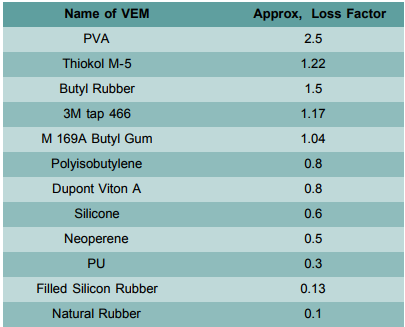Consider the generalised stress-strain relationship as follows: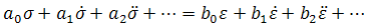For harmonic excitation at steady state applying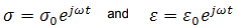We get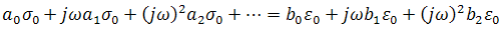Therefore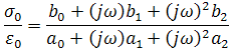This ratio could be denoted as complex Young's modulus E* such that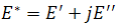where E' is the storage modulus and E" is the loss modulus. The loss factor n is expressed as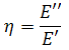Hence,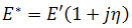Similarly, the shear modulus of VEM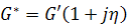and the bulk modulus of VEM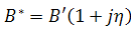The various modululli are interrelated as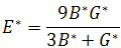Variation of the storage modulus and the loss factor of VEM with frequency and temperature are shown in Figs. 14.1a to 14.1d.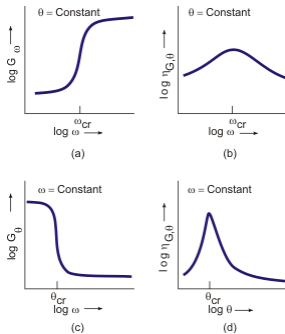Figure 14.1: Variation in storage modulus and the loss factor with frequency

The figures depict the following facts:
a. The shear modulus is low at low frequency and increase sharply beyond a critical frequency ωcr
b. The loss modulus also reaches its maxima at the same frequency
c. A reverse change is observed in the shear modulus with respect to temperature
d. Here also, the loss modulus increases sharply at a critical temperature

The critical frequency and temperature actually depict a phase change in the polymer. For frequency, the change occurs from rubbery to glassy phase and the reverse for temperature.

The frequency-dependence of the complex modulus we have just discussed can be explained through a linear viscoelastic model. For example, consider the simple, three-element model shown in the figure below: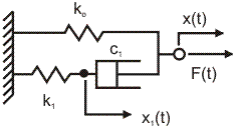Figure 14.2: 3 Element model

The stress-strain relation for this model is given by the following equation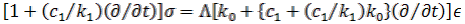(14.1)

where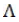is a geometric parameter.

Assuming a harmonic loading of frequency w, we substitute (jw) for the operator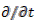in this equation. Then, we get the complex modulus as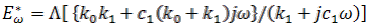(14.2)

Taking the real and imaginary parts of this equation, we obtain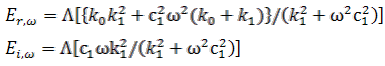(14.3)

It can be seen from eqns. (14.3) that the loss modulus Eiw has a maxima at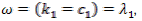where λ1 is the relaxation parameter of the viscous branch.

Offer running on EduRev: Apply code STAYHOME200 to get INR 200 off on our premium plan EduRev Infinity!

,

,

,

,

,

,

,

,

,

,

,

,

,

,

,

,

,

,

,

,

,

;